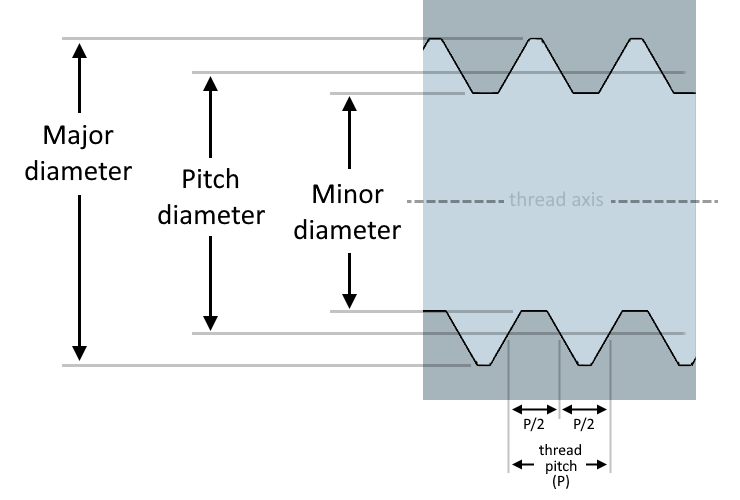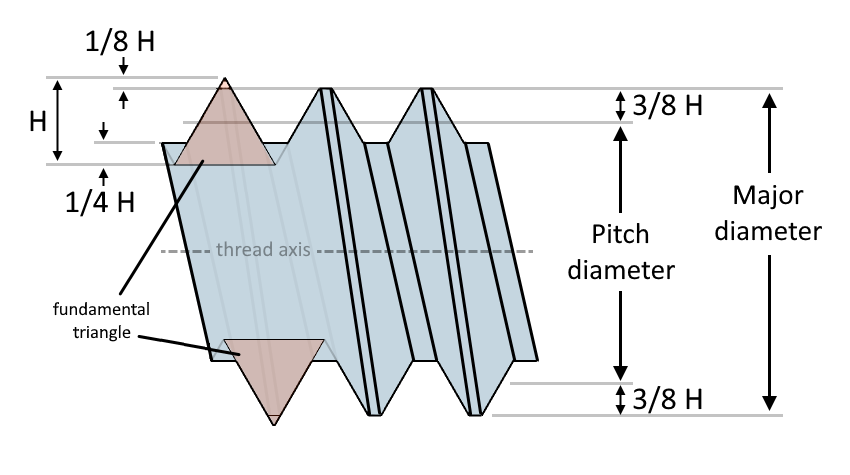# Pitch Diameter Calculator

Created by Kenneth Alambra
Reviewed by Wojciech Sas, PhD and Steven Wooding
Last updated: Feb 02, 2023

This pitch diameter calculator will help you find one of the essential dimensions of threaded fasteners – the pitch diameter. In this calculator, you will learn:

• What pitch diameter is;
• How to use the metric thread pitch diameter calculator;
• The different pitch diameter formulas; and
• How to calculate thread pitch diameter.

## What is pitch diameter?

Threads on threaded fasteners have three different diameters essential in determining the proper fit of bolts in nuts or bolts in threaded holes. These three diameters are the major diameter, minor diameter, and pitch diameter, as shown in the diagram below:We can also see in the diagram above that the thickness of the thread and the space between two threads are equal along the pitch diameter. At the same time, the sum of these distances also equates to the thread's pitch, hence the name pitch diameter. Learn more about the pitch of screw threads by checking out our thread pitch calculator.

Before we learn how to find a pitch diameter's value, let us first discuss how to use this pitch diameter calculator in the next section of this text.

🙋 Please note that this tool only works as an ISO Metric Thread pitch diameter calculator. That means all the calculations this tool performs are for 60º-angle threads under ISO Metric Standards only.

## How to use the metric thread pitch diameter calculator?

Let's say we want to calculate the pitch diameter of an $\text{M25 × 1.5 - 6g}$ bolt. Since this is a callout for a metric bolt thread, our first step is to:

1. Pick external thread dimensions for the option of what we want to calculate.
2. Next, we select $\small{1.5\ \text{mm}}$ for the thread pitch and enter $\small{25\ \text{mm}}$ for the Basic major diameter.
3. We then select $\small{6}$ and $\small{\text{g}}$ for the tolerance grade and tolerance position, respectively.

Upon completing these steps, we should already get the values for the basic pitch diameter of $\small{24.026\ \text{mm}}$, the maximum pitch diameter of $\small{23.994\ \text{mm}}$, and the minimum pitch diameter of $\small{23.850\ \text{mm}}$.

If you want to see the preliminary values used to obtain these pitch diameter values, go to the advanced mode of the calculator. Doing so will display the values for the fundamental deviation and tolerances, upper deviation ($\small{es}$) and pitch diameter tolerance ($\small{T_\text{d2}}$), and the fundamental triangle height ($\small{H}$) of the thread.

In the next section of this text, we'll discuss the different formulas we use in our pitch diameter calculator.

## Different pitch diameter formulas

From our discussion of how to use the pitch diameter calculator, we know that we need the values of the basic major diameter and the pitch of the thread we are investigating. Knowing those values, we can easily find the basic pitch diameter using this formula:

$d_2 = d - \left(3\times \sqrt{3} \times \frac{P}{8}\right)$

where:

• $d_2$Basic pitch diameter;
• $d$Basic major diameter; and
• $P$Thread pitch.

We derive this formula by substituting the value of the thread's fundamental triangle height, $H$, which is equal to $\small{(\sqrt{3}) \times \frac{P}{2}}$ into this equation $\small{d_2 = d - (2 \times \frac{3}{8}}) \times H$, where it means that $\small{\frac{3}{8}\ \text{of}\ H}$ gets deducted from both sides of the basic major diameter, as illustrated below:💡 An alternate equation to find pitch diameter is: $\small{d_2 = d - 0.6495 \times P}$. The $\small{3 \times \frac{\sqrt{3}}{8}}$ part of the previously given formula is equal to 0.6495190528 or approximately 0.6495.

Now that we know how to find the pitch diameter's base value, let us now figure out the maximum and minimum limits of the pitch diameter. Here are the equations that we use to find those values:

For external thread (bolt or screw) pitch diameter (d₂):

• $d_\text{2max} = d_2 + es$
• $d_\text{2min} = d_2 + es - T_\text{d2}$

• $D_\text{2max} = D_{2} + EI + T_\text{D2}$
• $D_\text{2min} = D_{2} + EI$

where:

• $es$ and $EI$fundamental deviations, upper and lower deviations, respectively; and
• $T_\text{d2}$ and $T_\text{D2}$thread pitch diameter tolerance for external and internal threads, respectively.

The fundamental deviations and tolerances are the minute allowances that we apply on the threads to give the threaded fasteners some wiggle room when they are threaded together. We can either have them fit very tightly or have more wiggle room, but we cannot file down threads such that they become very loose even at their recommended bolt torques. Explore the physics behind how much torque a bolt needs for a sufficient tightness with our bolt torque calculator.

We can calculate the fundamental deviations, $es$ and $EI$, depending on the thread's tolerance position:

• For e position: $\small{es = -(50 + 11 \times P) / 1000}$;
• For f position: $\small{es = -(30 + 11 \times P) / 1000}$ ;
• For g position: $\small{es = -(15 + 11 \times P) / 1000}$; and
• For h position: $\small{es = 0}$.

• For G position: $\small{EI = (15 + 11 \times P) / 1000}$; and
• For H position: $\small{EI = 0}$.

On the other hand, we determine the tolerance values using these equations for external and internal threads, respectively:

• $\small{T_\text{d2}(n) = k \times (90 \times P^{0.4} \times d^{0.1} / 1000)}$
• $\small{T_\text{D2}(n) = k \times (90 \times P^{0.4} \times d^{0.1} / 1000)}$

They are rather the same, but their multipliers, $\small{k}$, depend on the tolerance grade of the thread indicated by the value of $\small{n}$. For a tolerance grade of 6 for an external thread, we should use $\small{1.0}$ for the value of $\small{k}$. We can see the other values of $\small{k}$ in the table below:

n 3 k for Td2(n) for TD2(n) 0.50 — 0.63 0.85 0.80 1.06 1.00 1.32 1.25 1.70 1.60 2.12 2.00 —

## How to calculate thread pitch diameter example

Let's say we have an M30×2-6h bolt, and we want to determine its screw pitch diameter. We know that our bolt has a 30-mm basic major diameter and a 2-mm thread pitch given the thread callout. From there, we can already calculate our bolt's basic pitch diameter, as shown below:

\scriptsize \begin{align*} d_2 &= d - \left(3\times \sqrt{3} \times \frac{P}{8}\right)\\ &= 30\ \text{mm} - \left(3\times \sqrt{3} \times \frac{2\ \text{mm}}{8}\right)\\ &= 28.701\ \text{mm} \end{align*}

Since our bolt has a tolerance class of 6h, we can use the table in the previous section to find the multiplier $\small{k}$ to be equal to 1, and from the list of fundamental deviation formulas, we know that $\small{es = 0\ \text{mm}}$. We can then obtain the tolerance, $\small{T_{d2}}$ with this equation:

\scriptsize \begin{align*} T_\text{d2}(n) &= k \times \frac{(90 \times P^{0.4} \times d^{0.1})}{1000}\\\\ T_\text{d2}(6) &= 1 \times \frac{(90 \times 2\ \text{mm}^{0.4} \times 30\ \text{mm}^{0.1})}{1000}\\\\ &= (90 \times 1.31950 \times 1.40511) / 1000\\ &= 0.16687\ \text{mm} \end{align*}

Finally, we can solve for the maximum pitch diameter, as follows:

\scriptsize \begin{align*} d_\text{2max} &= d_2 + es\\ &= 28.701\ \text{mm} + 0\ \text{mm}\\ &= 28.701\ \text{mm} \end{align*}

And the minimum pitch diameter:

\scriptsize \begin{align*} d_\text{2min} &= d_2 + es - T_{d2}\\ &= 28.701\ \text{mm} + 0\ \text{mm} - 0.16687\ \text{mm}\\ &= 28.534\ \text{mm} \end{align*}

If you found this calculator useful, perhaps you'll also find our bolt circle calculator informational. That tool will help you estimate the location of holes in a circular pattern, especially when drilling bolt holes on a flange.

## FAQ

### What is the pitch diameter of a gear?

The pitch diameter of a gear is the diameter of the gear's pitch circle, which has a radius equal to the distance from the gear's center to its pitch point. The pitch point is the point wherein two gears interact with each other. Unlike in threaded fasteners, where external and internal threads should have the same thread pitch and pitch diameter to mate perfectly, gears can mate together even when they don't have the same pitch diameters as long as they have the same gear pitch.

### How do I find the pitch diameter?

Let's say you have a metric thread bolt with an outside diameter (or major diameter) of 20 mm and has threads that are 1.5 mm apart.

1. Multiply 1.5 mm by 0.6495 to get 0.9743 mm.
2. Subtract 0.9743 mm from the 20-mm outside diameter to obtain the pitch diameter of 19.0257 mm.

### How do I find the pitch diameter of an internal thread?

You can use the formula pitch diameter = major diameter - 0.6495 × thread pitch if you know the major diameter of the internal thread in question. On the other hand, you can also measure the minor diameter of an internal thread using a pair of calipers and then use this equation: pitch diameter = minor diameter + 0.4330 × thread pitch.

### What is the pitch diameter limits?

Pitch diameter limits are the maximum and minimum pitch diameters that a threaded fastener can have. Setting limits for thread diameters provides standard allowances for every manufactured thread depending on preferred tolerance. Exceeding these limits can lead to bolts and nuts of supposedly the same measurements to either loosen when fit together or not fit at all.

Kenneth Alambra
Option
Calculate...
Preliminary dimensions
1.5 mm
Basic major diameter
in
Tolerance class details
6
Tolerance position
g
Basic pitch diameter
in
Maximum pitch diameter
in
Minimum pitch diameter
in
People also viewed…

### BMR - Harris-Benedict equation

Harris-Benedict calculator uses one of the three most popular BMR formulas. Knowing your BMR (basal metabolic weight) may help you make important decisions about your diet and lifestyle.

### Car crash force

With this car crash calculator, you can find out how dangerous car crashes are.

### Concrete weight

Use the concrete weight calculator to see how much a specific volume of concrete weighs.

### Pipe volume

Use the pipe volume calculator to find the volume of a pipe and how much the liquid inside it weighs.